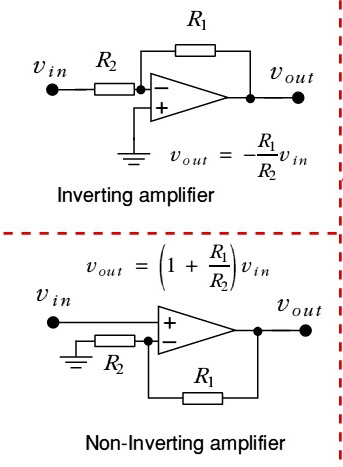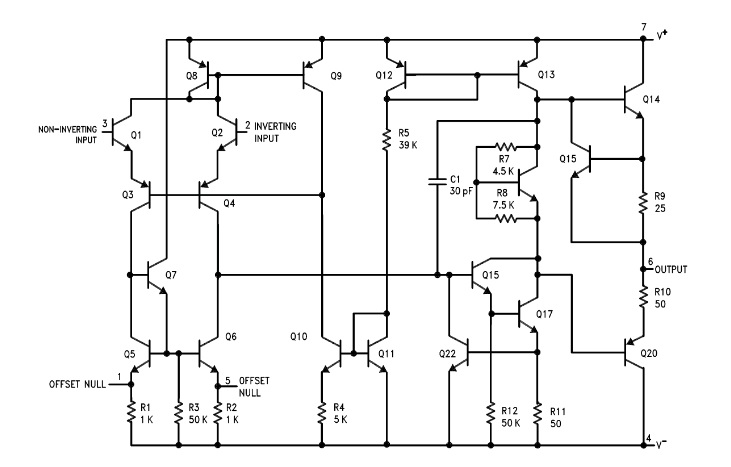## Sunday, February 14, 2016

### OpAmp - Why dual power supply?

Ok here is the circuit:Pin 7 is plus supply and pin 4 is minus supply. If the circuit is balanced the output at pin 6 will be half way between the voltage on pin 4 and 7. With a single supply the voltage would be half of supply. With dual supply it will still be half way so assuming V+=V- it would be 0 Volts. The key word in that statement was if the circuit is balanced. Now look at Q1 thru Q8 and accept that the circuit is a balanced bridge and the source is constant current. Because the circuit is balanced half of the current goes through both sides. Any off set in the inputs will cause the bridge to go off balance and cause a swing in output. Far practical purposes the circuit has an unlimited gain so the input differential required to produce a full swing in output is very small.

Now how is it used?

First example inverting amp. R1 and R2 ratio determine the gain. Input causes the output to swing which causes current to flow through R1 and R2. This current will self regulate at the point where the plus and minus inputs balance. The ground on the plus input will match with the ghost ground on the minus input and the circuit equilibrium is reached when the output is equal the ratio R1 to R2 times Vin.

Second example non inverting amp. Exact same as inverting amp with one exception. The output is balanced when the voltage drop across R2 equals the input in both case but in the inverting it is canceling the input and putting a ghost ground on the inverting input. In the non inverting circuit it must put a voltage on the inverting terminal equal the input.

With a balance dual supply the null output is 0 Volts. A single supply complicates matters because of the nature of the beast. The beast can be tamed but it requires extraordinary measures. Why complicate matters?
If you do want a single supply Mr Chichin wrote an article examining some of the pitfalls. It can be founf here.
http://www.analog.com/library/analogDialogue/archives/35-02/avoiding/avoiding.pdf